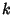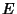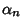## Simplex

The generalization of a tetrahedral region of space to-D. The boundary of a-simplex has0-faces (Vertices),1-faces (Edges), and-faces, whereis a Binomial Coefficient.

The simplex in 4-D is a regular Tetrahedronin which a pointalong the fourth dimension through the center ofis chosen so that. The 4-D simplex has Schläfli Symbol.Simplex 0 Point 1 Line Segment 2 Equilateral Triangular Plane Region 3 Tetrahedral Region 4 4-simplex

The regular simplex in-D withis denotedand has Schläfli Symbol.

See also Complex, Cross Polytope, Equilateral Triangle, Line Segment, Measure Polytope, Nerve, Point, Simplex Method, Tetrahedron

References

Eppstein, D. Triangles and Simplices.'' http://www.ics.uci.edu/~eppstein/junkyard/triangulation.html.